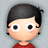#### Python Note

5月前 144

-*- coding: utf-8 -*-

from __future__ import print_function

print('python version %.2f' %(3.6) , end='' )

stock='tsmc'

price=220.5

print(' the %s price is %d' %(stock,price)  )

print(stock, price, sep=' = ')

print('%s: %-.4f, %05d' % ('RESULT', 3.14159, 42))

print('%s: %-.3f' % ('RESULT',3.14159), file=open('FILE','w'), end='' )

print( open('FILE','r').read() , ' 100%(from file) ' )

print('\\n*2= \n\n', end='')

import os

print(__name__)

print(                                                      __file__)

print(                           os.path.abspath(__file__))

print(os.path.dirname(os.path.abspath(__file__)))

---------------------------------------------- Results ----------------------------------------------------------------

python version 3.60 the tsmc price is 220

tsmc = 220.5

RESULT: 3.1416, 00042

RESULT: 3.142  100%(from file)

\n*2=

__main__

test.py

/twhome/cmliut/p/test.py

/twhome/cmliut/p

•4月前
引用 2

https://cizixs.com/2014/11/08/understand-wsgi/

•4月前
引用 3

Regular Expression

http://pjack1981.blogspot.com/2011/11/python-27-standard-library-regular.html

\d 就是 [0-9]                   \D 則是 [^0-9]

\s 就是 [ \t\n\r\f\v]            \S 則是 [^ \t\n\r\f\v]

\w 就是 [a-zA-Z0-9_]       \W 則是 [^a-zA-Z0-9_]

[] 是一堆字元的集合, 只要出現裡面任一字元, 就算符合

* 是重覆  0 ~ 無限多次

+ 是重覆 1 ~ 無限多次

? 是重覆 0 或 1 次

• re.compile 會回傳一個 pattern object，利用這個 pattern object 可以持續的分解一段文字，還滿好用的

• 要注意 raw string 及非 raw string 的差別，一般會直接使用 raw string，比較直覺
`# 第一個方案用一般  string 來找尋 '\n' 必須要輸入 "\\n"`
`>>> p  ``=` `re.``compile``(``'\\n'``, re.IGNORECASE)   `
`>>> p.findall(``"\np"``)`
`[``'\n'``]`
`>>> p.findall(``"\\np"``)`
`[]`
`# 第二個方案用 raw string， 就直接輸入 "\n" 就可以了，效果是一樣的`
`>>> p  ``=` `re.``compile``(r``'\n'``, re.IGNORECASE)`
`>>> p.findall(``"\np"``)`
`[``'\n'``]`
`>>> p.findall(``"\\np"``)`
`[]`

`>>> ``print` `p.findall(``"\np"``)[``0``]`

Compilation Flags

IGNORECASE, 這個應該很方便, 可以省去很多必須注意的小地方

MULTILINE, 可以自動把每一行分開解析

A | B 可以找尋 A 或 B, A 和 B 分別是一個 RE

^A 可以指定字串起始點必須要符合 A, A 是一個 RE

不過在集合 [] 內, ^ 是當反相的意思

A\$ 可以指定字串尾巴必須要符合 A, A 是一個 RE

\bS\b 用來指明要找尋的字串S前面或是後面必須要有分隔的字元

\BS\B 剛好是\b 的相反, 字串 S 的前面或是後面不可以是分隔的字元

() 用在區隔 group, 可以讓你一次在一個字串內找尋兩個 pattern, 甚至這兩個 pattern 是有交互作用的

(?P...) ,指定 group 的名字, (?P=name) 是舊的寫法

(?:...), 不指定 group 的名字, 只 mapping 但不抓回來, 這邊的寫法有點難以理解

`>>> m ``=` `re.match(``"([abc])+"``, ``"abc"``)`
`>>> m.groups()`
`(``'c'``,)`
`>>> m ``=` `re.match(``"(?:[abc])+"``, ``"abc"``)`
`>>> m.groups()`

`()`

(...)\1 可以用來指明第幾個 group, \1 表示要搜尋第一個 group

Splitting Strings

也可以利用 RE 來 split string, 符合的字串將會被消去, 然後分段整個字串

如果不想把符合的字串消去, 就必須加上 group

Search and Replace

符合的 pattern 可以用在 Replace Rule 當中, 這三種表示法都是同樣的意思 \1 = \g<1> = \g

*?、+?、?? 都是 Non-Greedy

•4月前
引用 4
•2月前
引用 5
python    -m    http.server
可虛擬一server給  tensorflow.js  抓檔案用
localhost:8000    或    127.0.0.1:8000    或    ip.ip.ip.ip:80000    可看